Courses

# Test: Enthalpy Of Neutralisation And Solution

## 19 Questions MCQ Test Chemistry for JEE | Test: Enthalpy Of Neutralisation And Solution

Description
This mock test of Test: Enthalpy Of Neutralisation And Solution for JEE helps you for every JEE entrance exam. This contains 19 Multiple Choice Questions for JEE Test: Enthalpy Of Neutralisation And Solution (mcq) to study with solutions a complete question bank. The solved questions answers in this Test: Enthalpy Of Neutralisation And Solution quiz give you a good mix of easy questions and tough questions. JEE students definitely take this Test: Enthalpy Of Neutralisation And Solution exercise for a better result in the exam. You can find other Test: Enthalpy Of Neutralisation And Solution extra questions, long questions & short questions for JEE on EduRev as well by searching above.
QUESTION: 1

### Direction (Q. Nos. 1-13) This section contains 13 multiple choice questions. Each question has four choices (a), (b), (c) and (d), out of which ONLY ONE option is correct. Q. If the enthalpy of HCI (g) and Cl- (aq) are - 92.30 kJ mol-1 and - 167.44 kJ mol-1 respectively, then ΔrH° for the following reaction is HCI (g) + aq → H+ (aq) + Cl- (aq)

Solution:

Enthalpy of reaction = ∑enthalpy of products - ∑enthalpy of reactants
Therefore
∆Hreaction = -167.44 - (- 92.30) (∆Hformation of H+ is 0)
= -75.14 kilo J / mole

QUESTION: 2

### The enthalpy of neutralisation of HS- (aq) is - 5.1 kJ mol-1. Thus, second ionisation energy of H2S is

Solution:

∆Hneutralisation =  ∆HH+ + OH- + ∆rHionisation
-5.1 = -57.3 + ∆Hionisation
∆Hionisation = 52.2 kJ mol-1

QUESTION: 3

### Given, ΔfH° of HCI (g) is - 22 . 10 kcal mol-1 and ΔSolutionH° (heat of solution) of HCI (g) is - 17.9 kcal mol-1. Thus, ΔfH ° of Cl- (aq) is

Solution:

½ H2(g) + ½ Cl2(g) → HCl(g) ∆fH° = -22.10 kcal mol-1  -----(I)
HCl(g) + H2O(l) → H+(aq) + Cl-(aq)     ∆solutionH° = -17.9 kcal mol-1 -----(II)
Let us add (I) and (II), we get
½ H2(g) + ½ Cl2(g) → H+(aq) + Cl-(aq) ∆rH° = -40.0 kcal mol-1
rH° = ∑∆Hproduct  -  ∑∆Hreactant = ∆fHCl- (since enthalpy of formation of H, H2 and Cl2 are 0)
fHCl- = -40.0

QUESTION: 4

Enthalpy of neutralisation of a weak dibasic acid by NaOH is - 24.0 kcal. Thus, enthalpy of ionisation of dibasic acid is

Solution:

Enthalpy of neutralisation = ∆HH+ + OH- → H2O + ∆Hionisation
-24.0 = -27.4 + ∆Hionisation   (since we have 2 moles of H+ and OH-, so enthalpy  will also double)
∆Hionisation = 3.4 kcal mol-1

QUESTION: 5

Consider the following reaction,

30 mL of 0.10 MNaOH (aq) + 10 mL of 0.10 M HCI (aq), enthalpy change is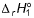.
On further adding 20 mL of 0.10 M HCI (aq), enthalpy change is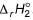. Thus, these values are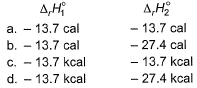Solution:
QUESTION: 6

In the following reactions,

I. 30 mL of 0.1 M Ba(OH)2(aq) + 30 mL of 0.1 M H2SO4 (aq), rise intemperature = ΔT1
II. 90 mL of 0.1 M Ba(OH)2(aq) + 90 mL of 0.1 M H2SO4 (aq), rise intemperature = ΔT2. Thus,

Solution:
QUESTION: 7

Given, CH3COOH(aq) → CH3COO(aq) + H+ (aq), ΔrH° = 0.005 kcal g-1

Enthalpy change when 1 mole of Ca(OH)2, a strong base, is completely neutralised by CH3COOH (aq) in dilute solution is

Solution:

CH3COOH → CH3COO- + H+
∆H = 0.3 kcal mol-1
H+ + OH- → H2O
∆H = -13.7 kcal mol-1
Equation needed
2CCH3COH + Ca(OH)2 → 2CH3COO + 2H2O
∆H = 2×EQN(I) + 2×EQN(II)
∆H = (0.3)2 + (-13.7)2 = -26.8 kcal mol-1

QUESTION: 8

For the reactions,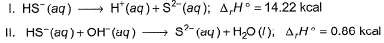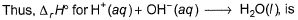Solution: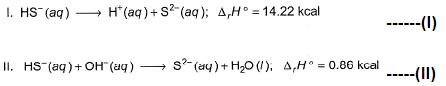For H+(aq) + OH-(aq) → H2O(l), we need to have -(I)+(II).
On doing the, we have ∆H = -14.22 + 0.66 = -13.56 kcal

QUESTION: 9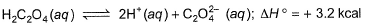Thus, heat of neutralisation of H2C2O4 (aq) is

Solution: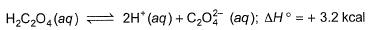We can say that enthalpy of neutralisation of H2C2O4 means that we have 2 moles of H+. So, for weak acid, enthalpy of neutralisation = heat released in neutralisation of H+ and OH- + enthalpy of ionisation.
∆Hneutralisation = 2 (-13.6) + 3.2 = -24.2 kcal mol-1

QUESTION: 10

Given,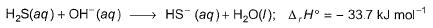Thus, for the reaction,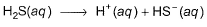ΔrH° is

Solution:

H2S(aq) + OH-(aq) → HS-(aq) + H2O(l)
(l) ∆Hr° = -33.7 kJ mol-1
We also have, H+(aq) + OH-(aq)  →  H2O(l)
(ll) ∆Hr° = -57.3 kJ mol-1
For the reaction,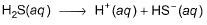we need (I) -(II)
So the ΔrH° be = -33.7 - (-57.3) = 23.6 kJ mol-1

QUESTION: 11

Hydration energies of Li+ and Cl- ions are - 499 and - 382 kJ mol-1 respectively. If lattice energy of LiCI is - 840 kJ mol-1 then heat of solution of LiCI is

Solution:

Li+ + H2O  →  Li+(aq.)
∆H1 = -499 kJ mol-1 ---------- (I)
Cl- + H2O →Cl-(aq)
∆H2 = -382 kJ mol-1 ---------- (II)
Li+ Cl→ LiCl(s)
∆H3 = -840 kJ mol-1---------- (III)
For r the heat of solution, we need the reaction,
LiCl(s) → Li+(aq.) + Cl-(aq)
This can be obtained by (I) + (II) - (III)
So the ∆H value be = -499 - 382 -(-840) = -41 kJ mol-1.

QUESTION: 12

Enthalpy change on mixing 100 mL of 1 M Ca(OH)2 with 100 mL of 1 M H2SO4 is

Solution:

For reaction of 1 mole of H+ and 1 mole of OH-, -13.6 kcal energy is released.
100 mL of 1 M Ca(OH)2 = moles = molarity volume = 0.1× 1 = 0.1
Moles of OH- = 0.2
100 mL of 1 M H2SO4=  moles = molarity volume = 0.1× 1 = 0.1
Moles of H+ = 0.2
SO 0.2 moles of H+ and OH- reacts.
Energy released, 0.2 ×13.6 =2.74 kcal.

QUESTION: 13

Taking each reactant in 1:1 molar ratio, enthalpy changes are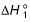and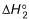in the following reactions.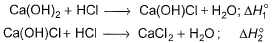andare (in kcal)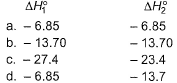Solution:

Since both reactions are acid base neutralization having strong acid-strong base and in 1:1 ratio of H+ and OH- ,so will have same enthalpy of reaction equal to-13.6 kcal.

QUESTION: 14

Direction (Q. Nos. 14) This sectionis based on statement I and Statement II. Select the correct answer from the code given below.
Q. Statement I : Based on the following thermochemical reactions :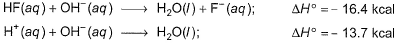Ionisation of HF(aq)is an exothermic reaction.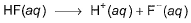Statement II : Enthalpy decrease in hydration of H+ (aq) and F- (aq) exceeds the ionisation energy of HF.

Solution: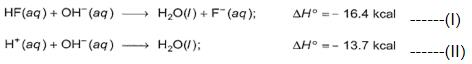On (I) - (II), we have
HF(aq)  →  H+(aq) + F-(aq)
∆H = -16.4-(-13.7) = -2.7 kcal
So, the ionisation of HF is an exothermic reaction. And so the statement I is true.
The reason behind HF being exothermic as the hydration energy of H+ and F- exceeds the lettuce enthalpy of HF and so overall the reaction becomes exothermic.

QUESTION: 15

Direction (Q. Nos. 15-16) This section contains a paragraph, wach describing  theory, experiments, data etc. three Questions related to paragraph have been  given.Each question have only one correct answer among the four given  ptions  (a),(b),(c),(d).

A quantity of 100 mL of 0.5 HCI is mixed with the 100 mL of 0.5 NaOH in a constant pressure calorimeter that has a heat capacity of 335 JK-1. Temperature of the mixture increased by 2.40 K. Density of the solution = 1g mL-1.

Q. Heat of solution due to mixing is

Solution:
QUESTION: 16

A quantity of 100 mL of 0.5 HCI is mixed with the 100 mL of 0.5 NaOH in a constant pressure calorimeter that has a heat capacity of 335 JK-1. Temperature of the mixture increased by 2.40 K. Density of the solution = 1g mL-1.

Q. Heat of neutralisation per mole of each reactant is

Solution:
*Answer can only contain numeric values
QUESTION: 17

Direction (Q. Nos. 17 - 19) This section contains 3 questions. when worked out will result in an integer from 0 to 9 (both inclusive).

Q. Lattice energy of NaCI (s) is - 788.0 kJ mol-1 and enthalpy of hydration is - 784.0 kJ mol-1. What is enthalpy change for the following reaction in kJ mol-1?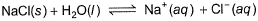Solution:
*Answer can only contain numeric values
QUESTION: 18

When 4.0 mL of 2.0 N solution of weak acid is neutralised by a dilute aqueous solution of sodium hydroxide, 64 cal of heat is liberated. What is the heat of neutralisation in cal/milliequivalent?

Solution:

Milliequivalents of the acid taken = 4×2 =8.
neutralization of 8 meq liberated 64 cal heat.
So the heat of neutralization is 64/8 = 8cal/meq.

*Answer can only contain numeric values
QUESTION: 19

Heat of neutralisation of a polybasic acid by a strong base is - 54.8 kcal mol-1? What is basicity of the acid?

Solution:

For reaction of 1 mole of H+ and one mole of OH-, -13.6 kcal energy is released. As we have energy released = -54.8,
Therefore -13.6×nH+ = -54.8
nH+ = 4
So, the basicity of acid given here is 4.